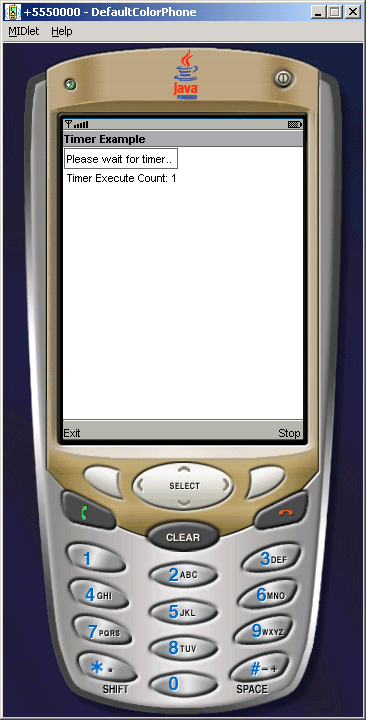Tutorials

# J2ME Timer MIDlet Example

This Example shows how to use of timer class.

This Example shows how to use of timer class.

# J2ME Timer MIDlet Example

This Example shows how to use of timer class. In this example we are using the Timer class to create the time of execution  of application and the TimerTask class is used to create the counter of timer which is executed after 5000 ms as below:

 ```private class TestTimerTask extends TimerTask{   public final void run(){   form.append("Timer Execute Count: " + ++count + "\n");   } }```

in the above source code we execute the counter of the timer on the mobile window as follows:TimerMIDlet.java

 ``` import java.util.*; import javax.microedition.midlet.*; import javax.microedition.lcdui.*; public class TimerMIDlet extends MIDlet implements CommandListener{   private Display display;   private Form form;   private Command exit, stop;    private Timer timer;    private TestTimerTask task;    private int count = 0;    public TimerMIDlet(){   display = Display.getDisplay(this);     form = new Form("Timer Example");   exit = new Command("Exit", Command.EXIT, 1);   stop= new Command("Stop", Command.STOP, 2);   form.append("Please wait for timer.. \n");   form.addCommand(exit);   form.addCommand(stop);   form.setCommandListener(this);     }     public void startApp (){   timer = new Timer();   task = new TestTimerTask();   timer.schedule(task,5000);   display.setCurrent(form);   }     public void pauseApp (){ }   public void destroyApp (boolean unconditional){   notifyDestroyed();   }     public void commandAction(Command c, Displayable d){   String label = c.getLabel();   if (label.equals("Stop")){   timer.cancel();   }else if (label.equals("Exit")) {   destroyApp(true);   }   }   private class TestTimerTask extends TimerTask{   public final void run(){   form.append("Timer Execute Count: " + ++count + "\n");   }   } }```